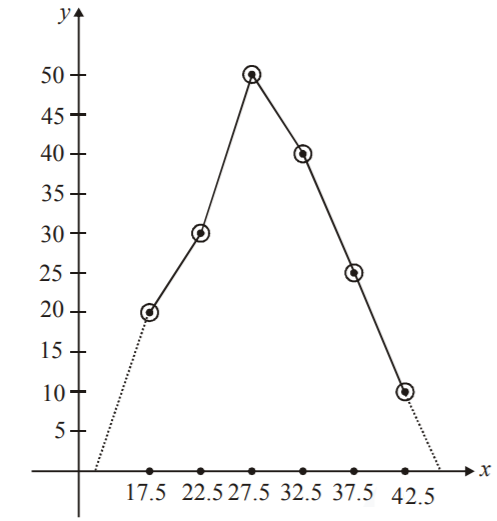# Maharashtra (MSBSHSE) SSC Board Question Paper For Class 10 Maths Paper 2 (Algebra) 2018 In PDF

## Maharashtra SSC Maths Algebra (Paper 2) Exam Question Paper 2018 with Solutions – Free Download

MSBSHSE (Maharashtra board) Public Exam Class 10 Maths 2018 Algebra Question Paper with solutions can be accessed at BYJU’S. Students can also get two papers of MSBSHSE Maths question papers for each year in a downloadable format. They can access solutions of all previous years’ SSC Maths question papers, which are prepared by the expert faculty at BYJU’S. Maharashtra board divided the whole syllabus of Class 10 Maths into two parts, hence the annual exam of MSBSHSE contains two papers, each year. All these SSC question papers and solutions will help the students in scoring full marks in the examination.

### MSBSHSE Class 10 Mathematics Question Paper 2018 Algebra Paper with Solutions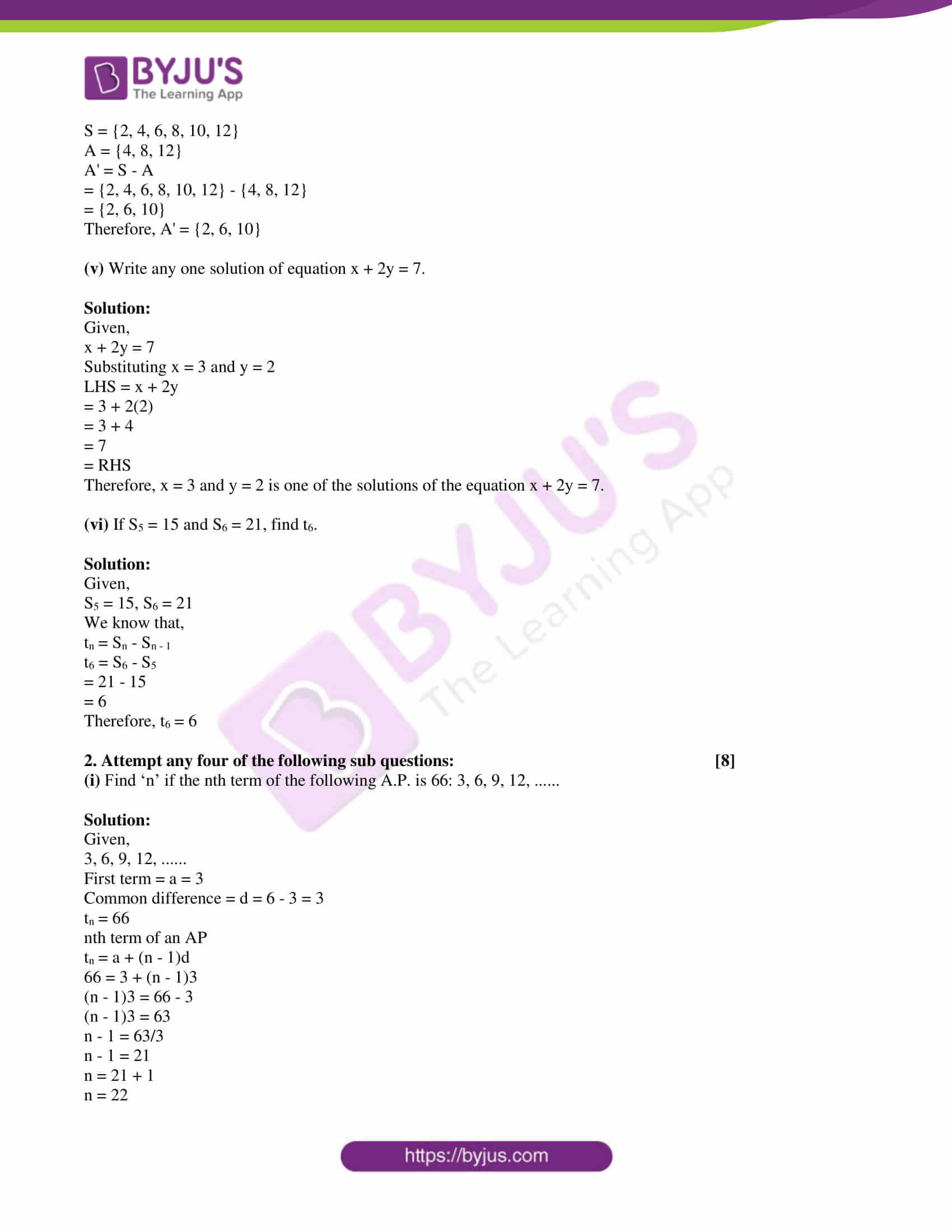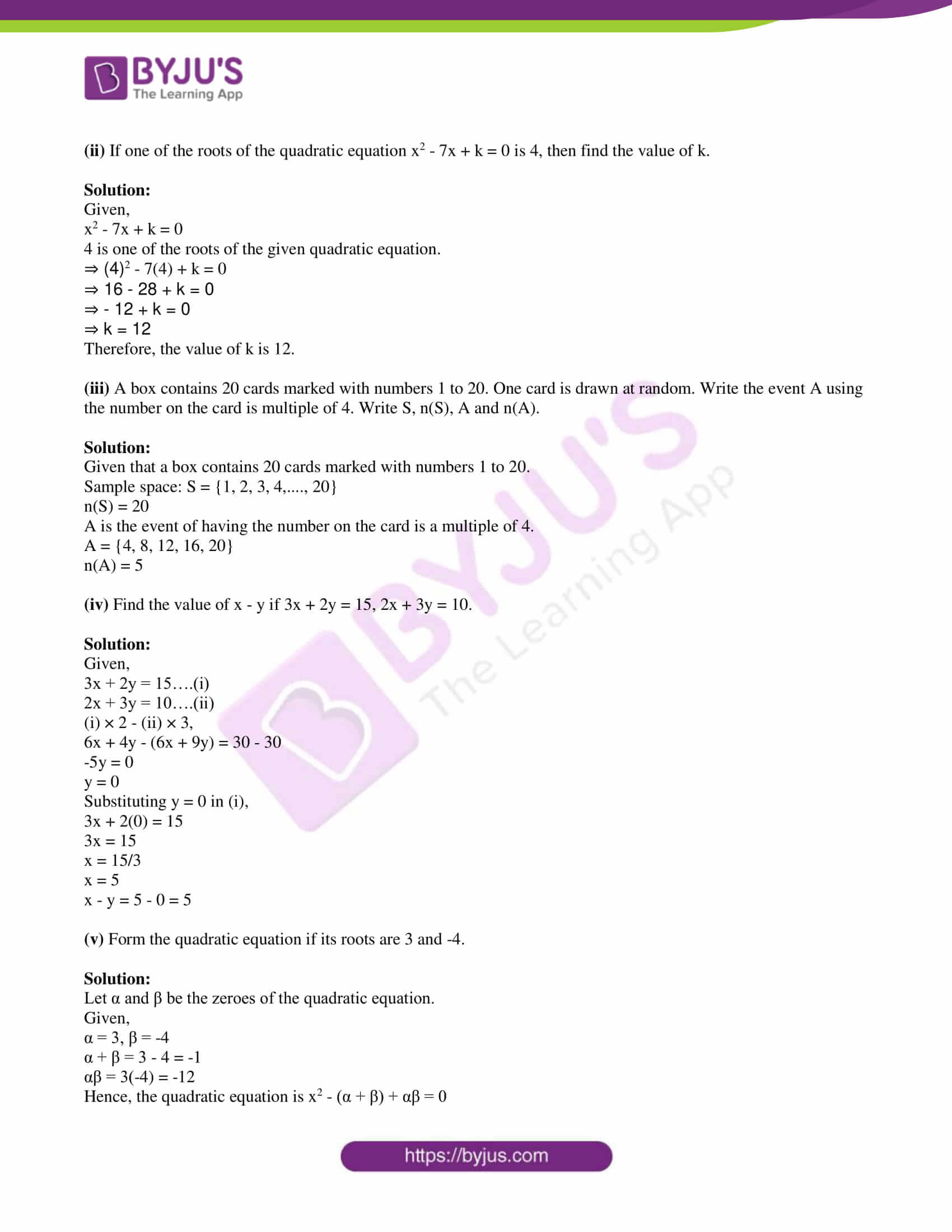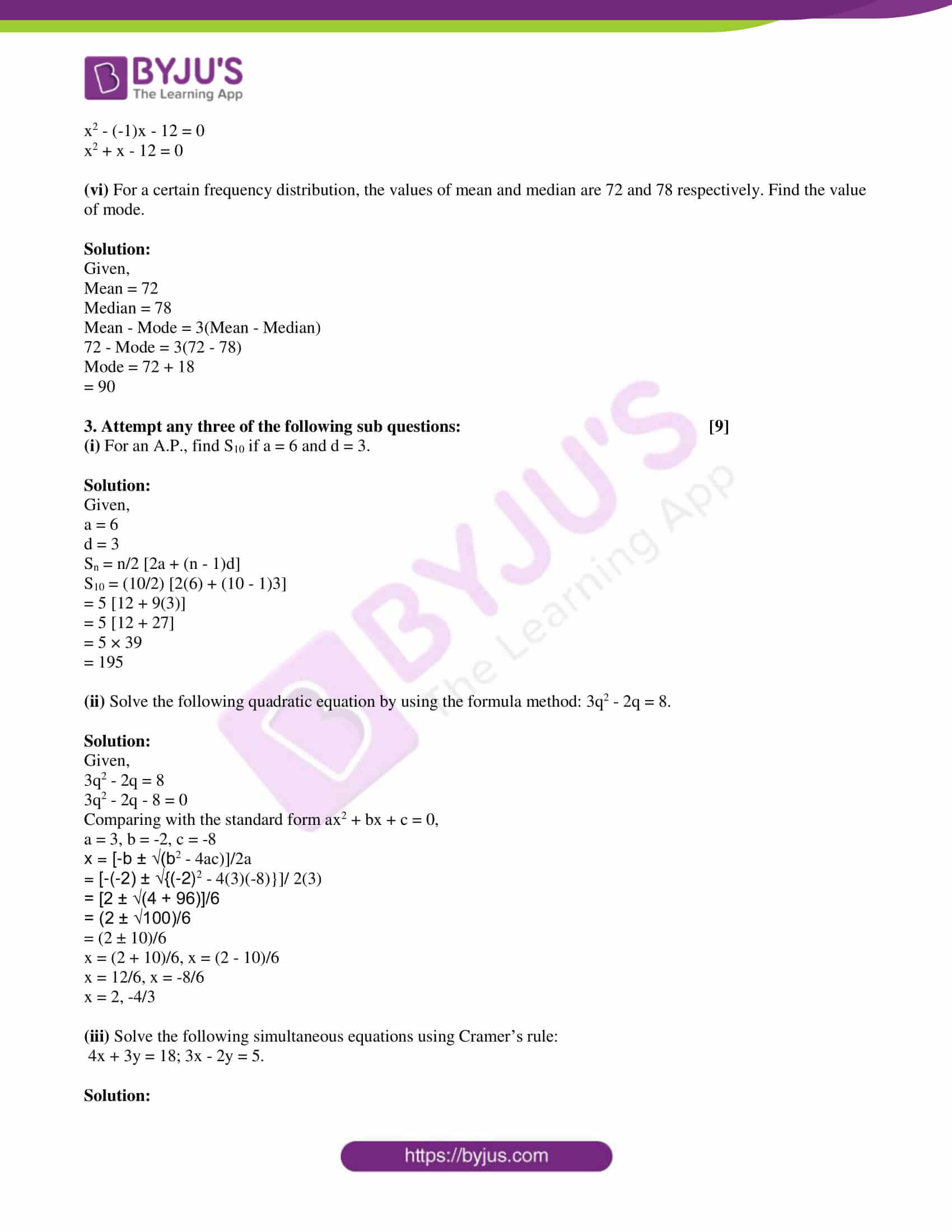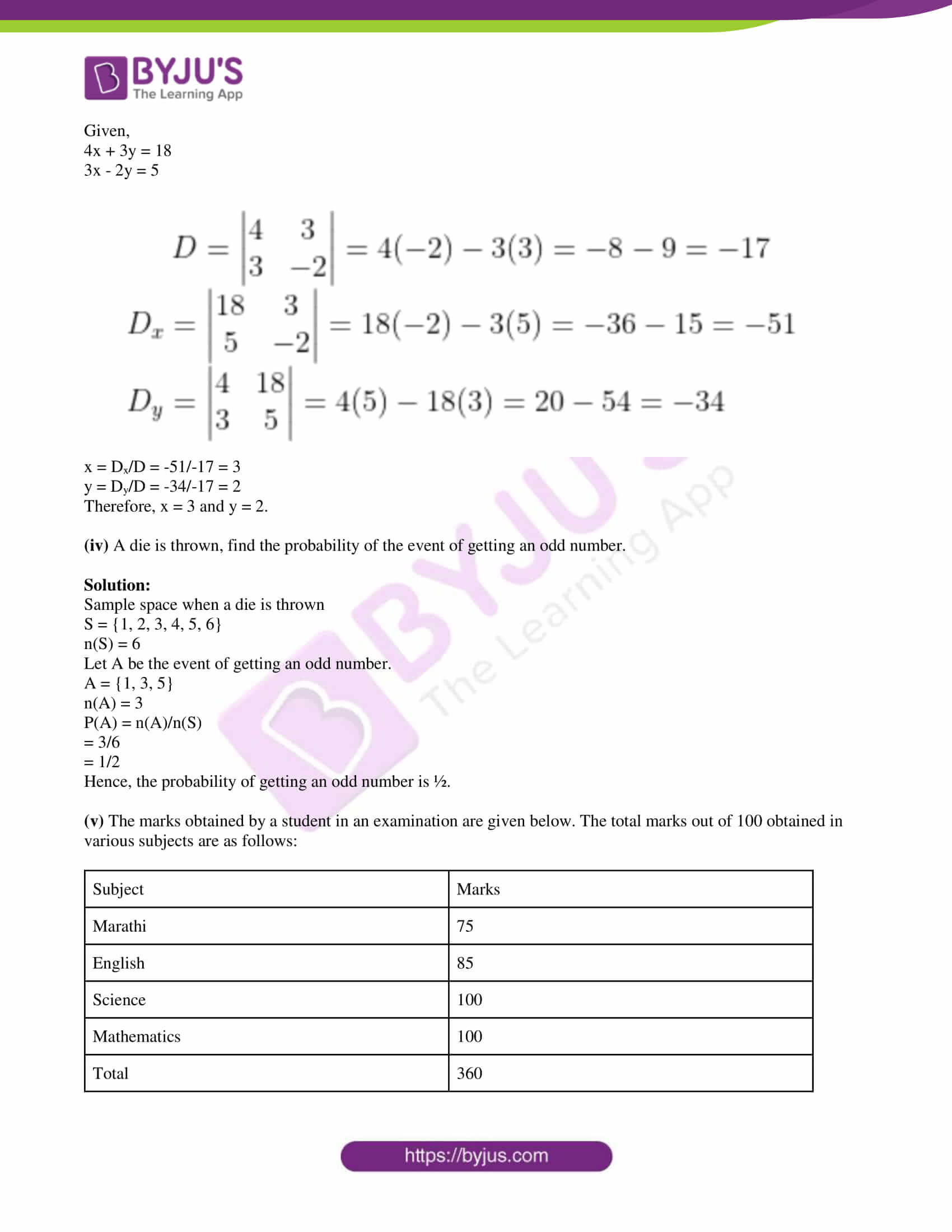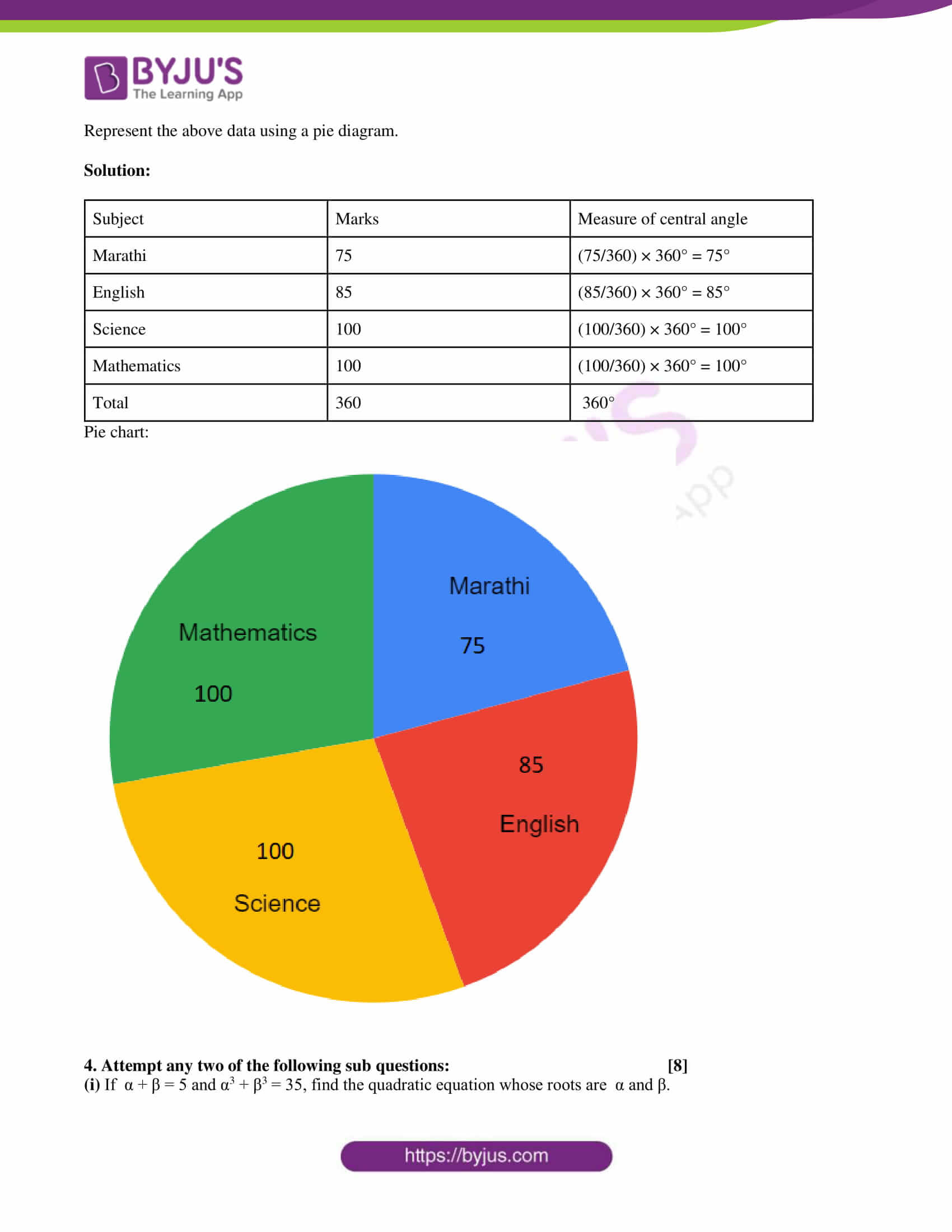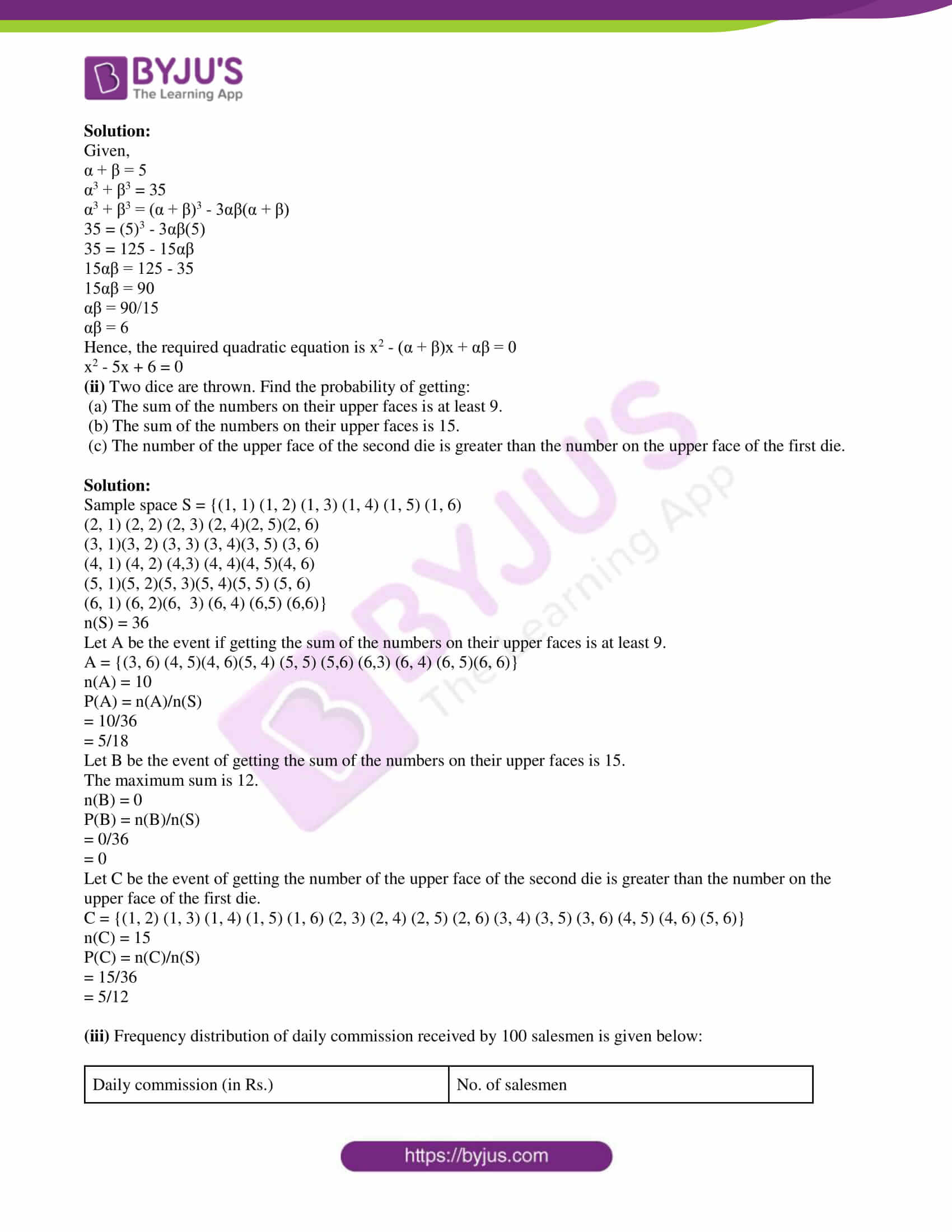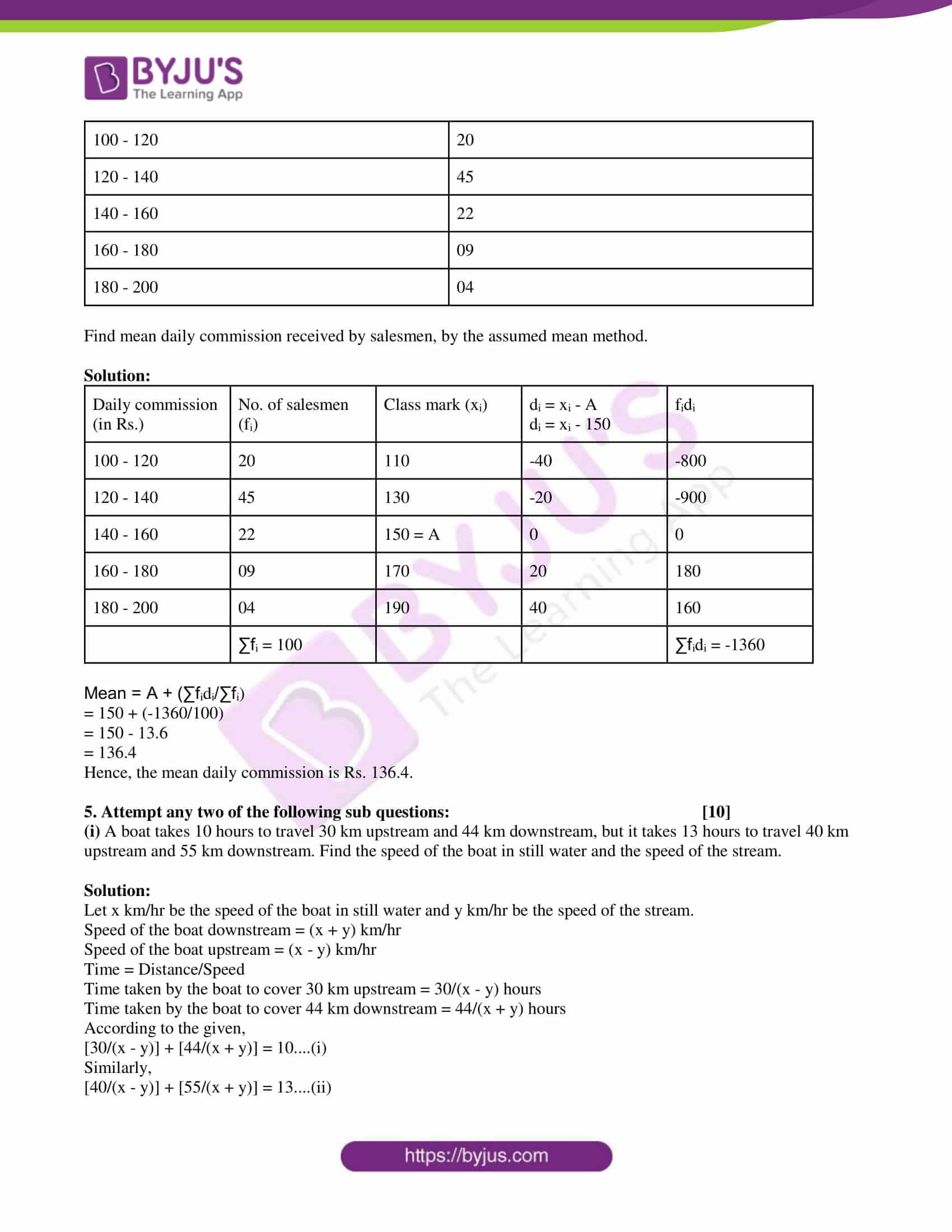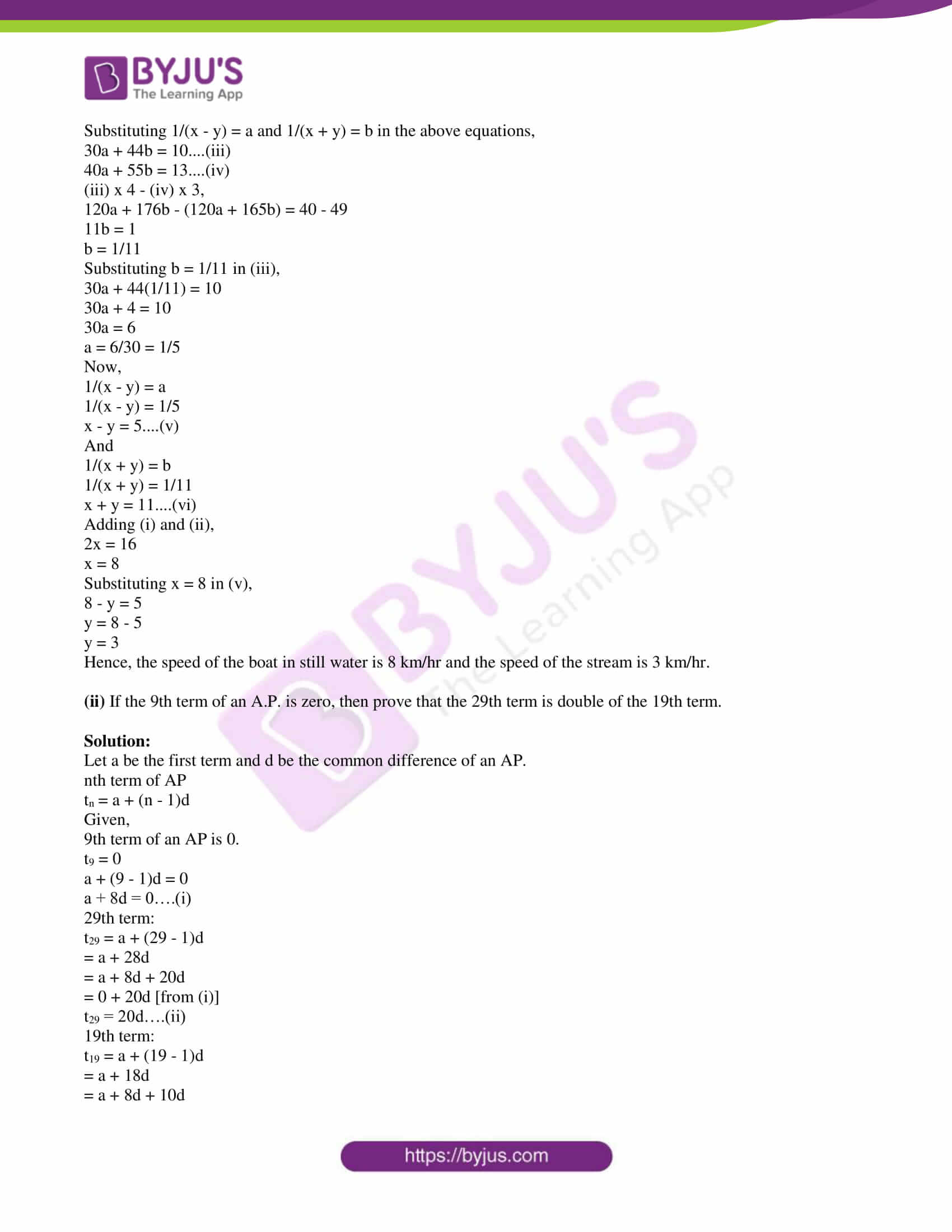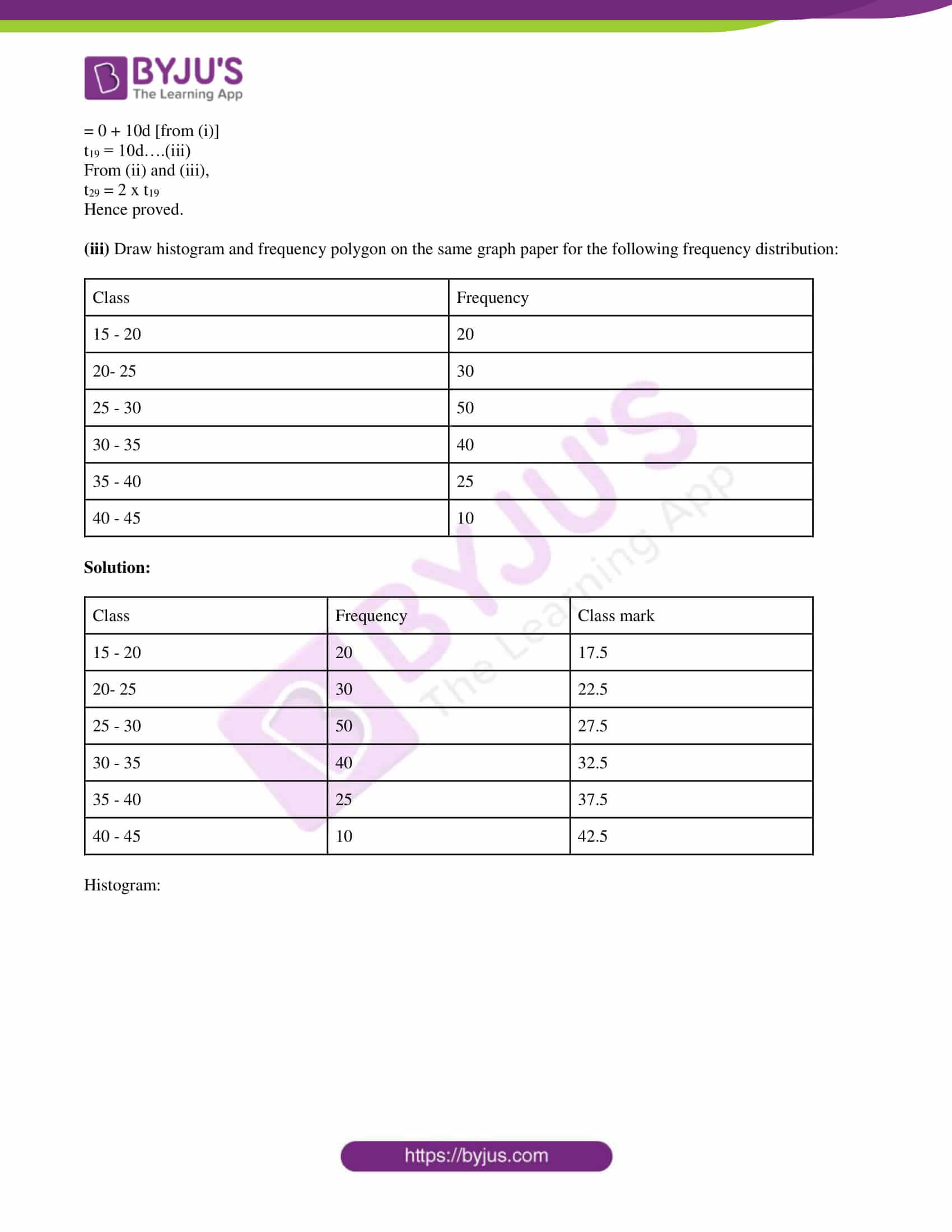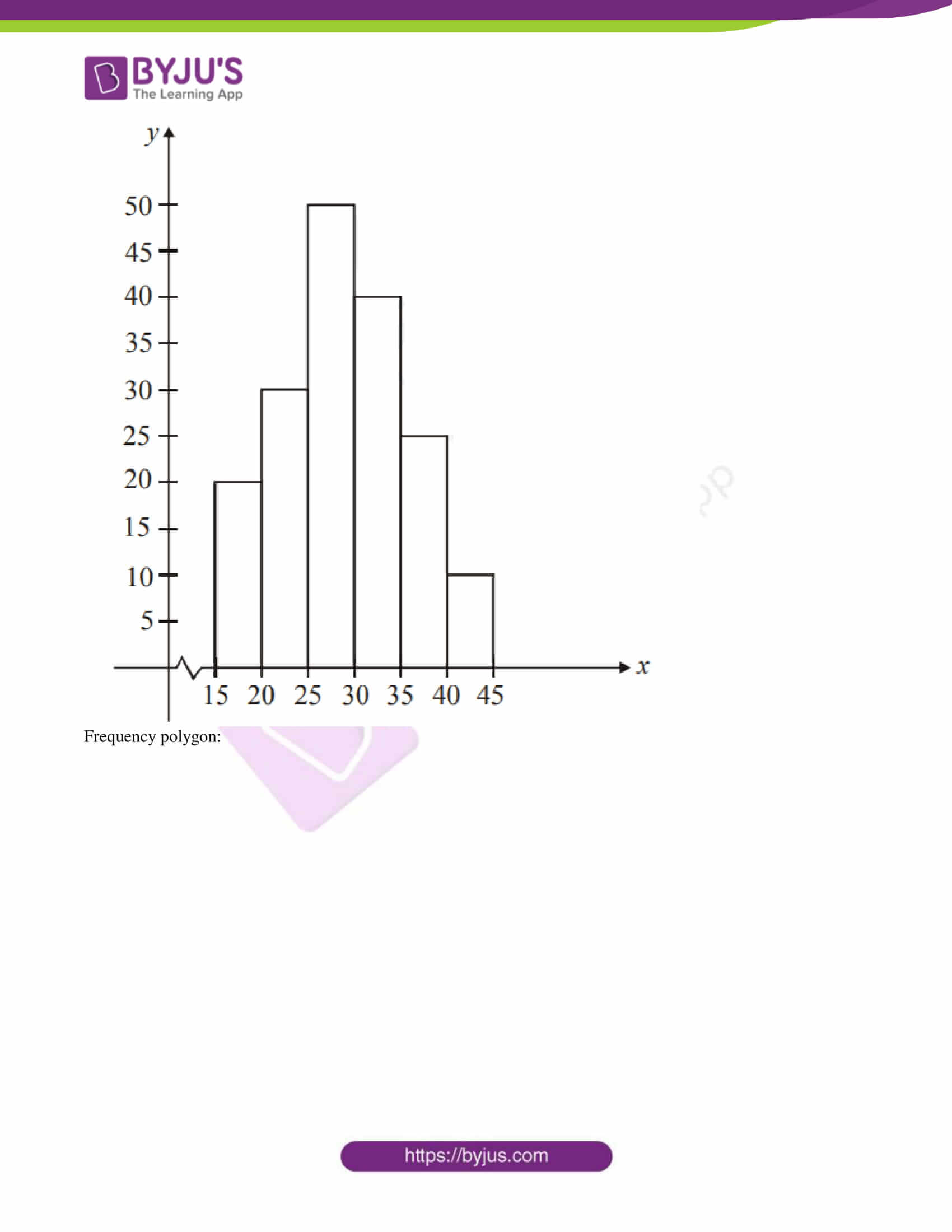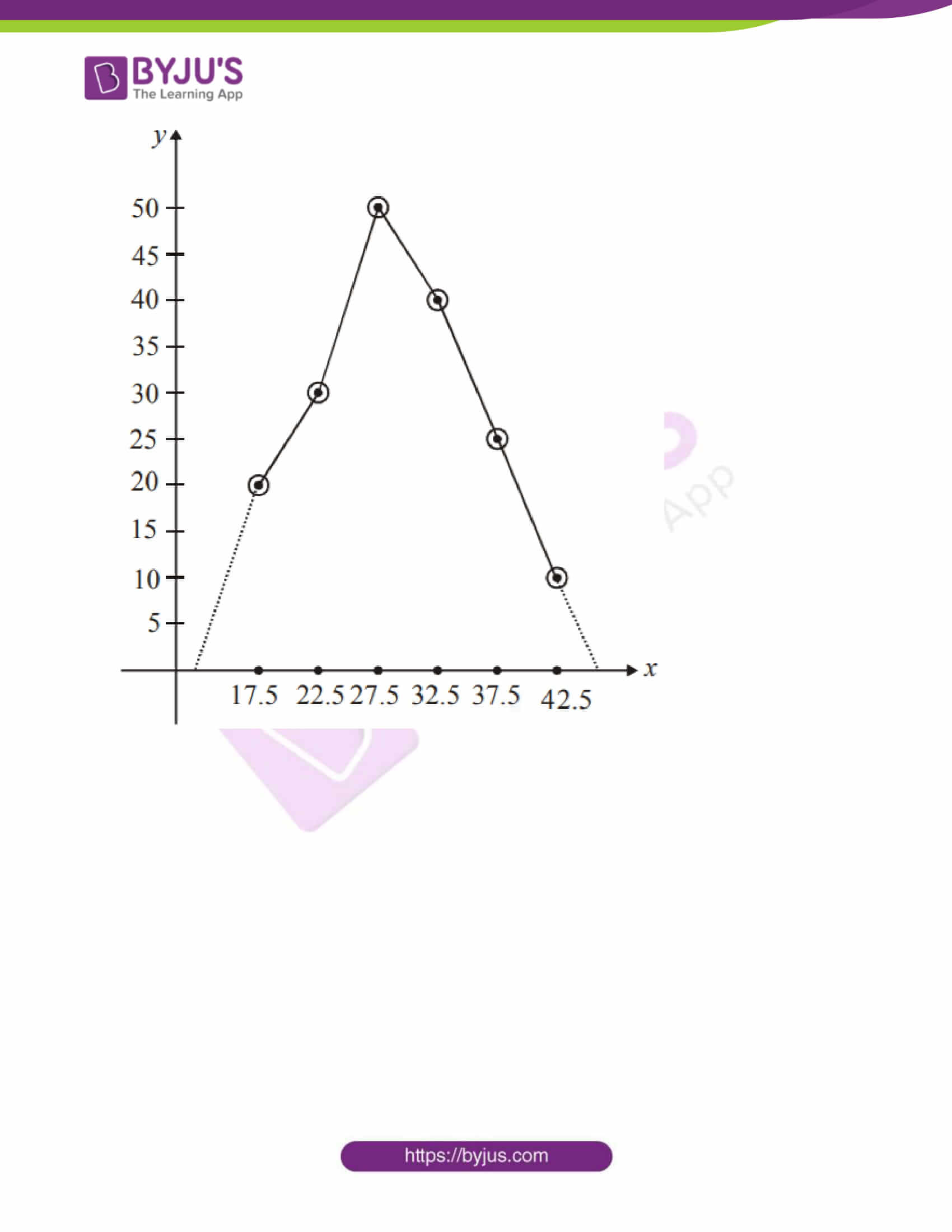PART – A

1. Attempt any five of the following subquestions: 

(i) Find the next two terms of an A.P. 4, 9, 14,…….

Solution:

Given AP:

4, 9, 14,…..

First term = a = 4

Common difference = d = 9 – 4 = 5

Fourth term = a + 3d = 4 + 3(5) = 4 + 15 = 19

Fifth term = a + 4d = 4 + 4(5) = 4 + 20 = 24

Hence, the next two terms of the given AP are 19 and 24.

(ii) State whether the given equation is quadratic or not. Give reason.

(5/4)m2 – 7 = 0

Solution:

Given,

(5/4)m2 – 7 = 0

Here, m = variable

Highest degree of the variable = 2

By comparing with the standard form ax2 + bx + c = 0,

a = 5/4, b = 0, c = -7

Thus, a, b, c are real numbers and a ≠ 0.

Hence, the given equation is a quadratic equation in variable m.

(iii) If Dx = 25, D = 5 are the values of the determinants for certain simultaneous equations in x and y, find x.

Solution:

Given,

Dx = 25, D = 5

By Cramer’s rule,

x = Dx/D

= 25/5

= 5

Therefore, x = 5

(iv) If S = {2, 4, 6, 8, 10, 12} and A = {4, 8, 12}, find A’.

Solution:

Given,

S = {2, 4, 6, 8, 10, 12}

A = {4, 8, 12}

A’ = S – A

= {2, 4, 6, 8, 10, 12} – {4, 8, 12}

= {2, 6, 10}

Therefore, A’ = {2, 6, 10}

(v) Write any one solution of equation x + 2y = 7.

Solution:

Given,

x + 2y = 7

Substituting x = 3 and y = 2

LHS = x + 2y

= 3 + 2(2)

= 3 + 4

= 7

= RHS

Therefore, x = 3 and y = 2 is one of the solutions of the equation x + 2y = 7.

(vi) If S5 = 15 and S6 = 21, find t6.

Solution:

Given,

S5 = 15, S6 = 21

We know that,

tn = Sn – Sn – 1

t6 = S6 – S5

= 21 – 15

= 6

Therefore, t6 = 6

2. Attempt any four of the following subquestions: 

(i) Find ‘n’ if the nth term of the following A.P. is 66: 3, 6, 9, 12, ……

Solution:

Given,

3, 6, 9, 12, ……

First term = a = 3

Common difference = d = 6 – 3 = 3

tn = 66

nth term of an AP

tn = a + (n – 1)d

66 = 3 + (n – 1)3

(n – 1)3 = 66 – 3

(n – 1)3 = 63

n – 1 = 63/3

n – 1 = 21

n = 21 + 1

n = 22

(ii) If one of the roots of the quadratic equation x2 – 7x + k = 0 is 4, then find the value of k.

Solution:

Given,

x2 – 7x + k = 0

4 is one of the roots of the given quadratic equation.

⇒ (4)2 – 7(4) + k = 0

⇒ 16 – 28 + k = 0

⇒ – 12 + k = 0

⇒ k = 12

Therefore, the value of k is 12.

(iii) A box contains 20 cards marked with numbers 1 to 20. One card is drawn at random. Write the event A using the number on the card is multiple of 4. Write S, n(S), A, and n(A).

Solution:

Given that a box contains 20 cards marked with numbers 1 to 20.

Sample space: S = {1, 2, 3, 4,…., 20}

n(S) = 20

A is the event of having the number on the card is a multiple of 4.

A = {4, 8, 12, 16, 20}

n(A) = 5

(iv) Find the value of x – y if 3x + 2y = 15, 2x + 3y = 10.

Solution:

Given,

3x + 2y = 15….(i)

2x + 3y = 10….(ii)

(i) × 2 – (ii) × 3,

6x + 4y – (6x + 9y) = 30 – 30

-5y = 0

y = 0

Substituting y = 0 in (i),

3x + 2(0) = 15

3x = 15

x = 15/3

x = 5

x – y = 5 – 0 = 5

(v) Form the quadratic equation if its roots are 3 and -4.

Solution:

Let α and β be the zeroes of the quadratic equation.

Given,

α = 3, β = -4

α + β = 3 – 4 = -1

αβ = 3(-4) = -12

Hence, the quadratic equation is x2 – (α + β) + αβ = 0

x2 – (-1)x – 12 = 0

x2 + x – 12 = 0

(vi) For a certain frequency distribution, the values of mean and median are 72 and 78 respectively. Find the value of mode.

Solution:

Given,

Mean = 72

Median = 78

Mean – Mode = 3(Mean – Median)

72 – Mode = 3(72 – 78)

Mode = 72 + 18

= 90

3. Attempt any three of the following subquestions: 

(i) For an A.P., find S10 if a = 6 and d = 3.

Solution:

Given,

a = 6

d = 3

Sn = n/2 [2a + (n – 1)d]

S10 = (10/2) [2(6) + (10 – 1)3]

= 5 [12 + 9(3)]

= 5 [12 + 27]

= 5 × 39

= 195

(ii) Solve the following quadratic equation by using the formula method: 3q2 – 2q = 8.

Solution:

Given,

3q2 – 2q = 8

3q2 – 2q – 8 = 0

Comparing with the standard form ax2 + bx + c = 0,

a = 3, b = -2, c = -8

x = [-b ± √(b2 – 4ac)]/2a

= [-(-2) ± √{(-2)2 – 4(3)(-8)}]/ 2(3)

= [2 ± √(4 + 96)]/6

= (2 ± √100)/6

= (2 ± 10)/6

x = (2 + 10)/6, x = (2 – 10)/6

x = 12/6, x = -8/6

x = 2, -4/3

(iii) Solve the following simultaneous equations using Cramer’s rule:

4x + 3y = 18; 3x – 2y = 5.

Solution:

Given,

4x + 3y = 18

3x – 2y = 5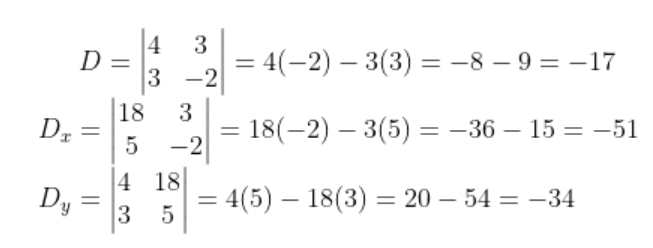x = Dx/D = -51/-17 = 3

y = Dy/D = -34/-17 = 2

Therefore, x = 3 and y = 2.

(iv) A die is thrown, find the probability of the event of getting an odd number.

Solution:

Sample space when a die is thrown

S = {1, 2, 3, 4, 5, 6}

n(S) = 6

Let A be the event of getting an odd number.

A = {1, 3, 5}

n(A) = 3

P(A) = n(A)/n(S)

= 3/6

= 1/2

Hence, the probability of getting an odd number is ½.

(v) The marks obtained by a student in an examination are given below. The total marks out of 100 obtained in various subjects are as follows:

 Subject Marks Marathi 75 English 85 Science 100 Mathematics 100 Total 360

Represent the above data using a pie diagram.

Solution:

 Subject Marks Measure of central angle Marathi 75 (75/360) × 360° = 75° English 85 (85/360) × 360° = 85° Science 100 (100/360) × 360° = 100° Mathematics 100 (100/360) × 360° = 100° Total 360 360°

Pie chart: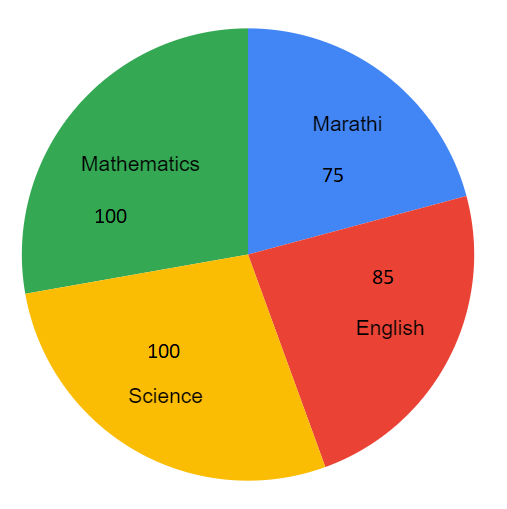4. Attempt any two of the following subquestions: 

(i) If α + β = 5 and α3 + β3 = 35, find the quadratic equation whose roots are α and β.

Solution:

Given,

α + β = 5

α3 + β3 = 35

α3 + β3 = (α + β)3 – 3αβ(α + β)

35 = (5)3 – 3αβ(5)

35 = 125 – 15αβ

15αβ = 125 – 35

15αβ = 90

αβ = 90/15

αβ = 6

Hence, the required quadratic equation is x2 – (α + β)x + αβ = 0

x2 – 5x + 6 = 0

(ii) Two dice are thrown. Find the probability of getting:

(a) The sum of the numbers on their upper faces is at least 9.

(b) The sum of the numbers on their upper faces is 15.

(c) The number of the upper face of the second die is greater than the number on the upper face of the first die.

Solution:

Sample space S = {(1, 1) (1, 2) (1, 3) (1, 4) (1, 5) (1, 6)

(2, 1) (2, 2) (2, 3) (2, 4)(2, 5)(2, 6)

(3, 1)(3, 2) (3, 3) (3, 4)(3, 5) (3, 6)

(4, 1) (4, 2) (4,3) (4, 4)(4, 5)(4, 6)

(5, 1)(5, 2)(5, 3)(5, 4)(5, 5) (5, 6)

(6, 1) (6, 2)(6, 3) (6, 4) (6,5) (6,6)}

n(S) = 36

Let A be the event if getting the sum of the numbers on their upper faces is at least 9.

A = {(3, 6) (4, 5)(4, 6)(5, 4) (5, 5) (5,6) (6,3) (6, 4) (6, 5)(6, 6)}

n(A) = 10

P(A) = n(A)/n(S)

= 10/36

= 5/18

Let B be the event of getting the sum of the numbers on their upper faces is 15.

The maximum sum is 12.

n(B) = 0

P(B) = n(B)/n(S)

= 0/36

= 0

Let C be the event of getting the number of the upper face of the second die is greater than the number on the upper face of the first die.

C = {(1, 2) (1, 3) (1, 4) (1, 5) (1, 6) (2, 3) (2, 4) (2, 5) (2, 6) (3, 4) (3, 5) (3, 6) (4, 5) (4, 6) (5, 6)}

n(C) = 15

P(C) = n(C)/n(S)

= 15/36

= 5/12

(iii) Frequency distribution of daily commission received by 100 salesmen is given below:

 Daily commission (in Rs.) No. of salesmen 100 – 120 20 120 – 140 45 140 – 160 22 160 – 180 09 180 – 200 04

Find mean daily commission received by salesmen, by the assumed mean method.

Solution:

 Daily commission (in Rs.) No. of salesmen (fi) Class mark (xi) di = xi – A di = xi – 150 fidi 100 – 120 20 110 -40 -800 120 – 140 45 130 -20 -900 140 – 160 22 150 = A 0 0 160 – 180 09 170 20 180 180 – 200 04 190 40 160 ∑fi = 100 ∑fidi = -1360

Mean = A + (∑fidi/∑fi)

= 150 + (-1360/100)

= 150 – 13.6

= 136.4

Hence, the mean daily commission is Rs. 136.4.

5. Attempt any two of the following subquestions: 

(i) A boat takes 10 hours to travel 30 km upstream and 44 km downstream, but it takes 13 hours to travel 40 km upstream and 55 km downstream. Find the speed of the boat in still water and the speed of the stream.

Solution:

Let x km/hr be the speed of the boat in still water and y km/hr be the speed of the stream.

Speed of the boat downstream = (x + y) km/hr

Speed of the boat upstream = (x – y) km/hr

Time = Distance/Speed

Time taken by the boat to cover 30 km upstream = 30/(x – y) hours

Time taken by the boat to cover 44 km downstream = 44/(x + y) hours

According to the given,

[30/(x – y)] + [44/(x + y)] = 10….(i)

Similarly,

[40/(x – y)] + [55/(x + y)] = 13….(ii)

Substituting 1/(x – y) = a and 1/(x + y) = b in the above equations,

30a + 44b = 10….(iii)

40a + 55b = 13….(iv)

(iii) x 4 – (iv) x 3,

120a + 176b – (120a + 165b) = 40 – 49

11b = 1

b = 1/11

Substituting b = 1/11 in (iii),

30a + 44(1/11) = 10

30a + 4 = 10

30a = 6

a = 6/30 = 1/5

Now,

1/(x – y) = a

1/(x – y) = 1/5

x – y = 5….(v)

And

1/(x + y) = b

1/(x + y) = 1/11

x + y = 11….(vi)

2x = 16

x = 8

Substituting x = 8 in (v),

8 – y = 5

y = 8 – 5

y = 3

Hence, the speed of the boat in still water is 8 km/hr and the speed of the stream is 3 km/hr.

(ii) If the 9th term of an A.P. is zero, then prove that the 29th term is double of the 19th term.

Solution:

Let a be the first term and d be the common difference of an AP.

nth term of AP

tn = a + (n – 1)d

Given,

9th term of an AP is 0.

t9 = 0

a + (9 – 1)d = 0

a + 8d = 0….(i)

29th term:

t29 = a + (29 – 1)d

= a + 28d

= a + 8d + 20d

= 0 + 20d [from (i)]

t29 = 20d….(ii)

19th term:

t19 = a + (19 – 1)d

= a + 18d

= a + 8d + 10d

= 0 + 10d [from (i)]

t19 = 10d….(iii)

From (ii) and (iii),

t29 = 2 x t19

Hence proved.

(iii) Draw histogram and frequency polygon on the same graph paper for the following frequency distribution:

 Class Frequency 15 – 20 20 20- 25 30 25 – 30 50 30 – 35 40 35 – 40 25 40 – 45 10

Solution:

 Class Frequency Classmark 15 – 20 20 17.5 20- 25 30 22.5 25 – 30 50 27.5 30 – 35 40 32.5 35 – 40 25 37.5 40 – 45 10 42.5

Histogram: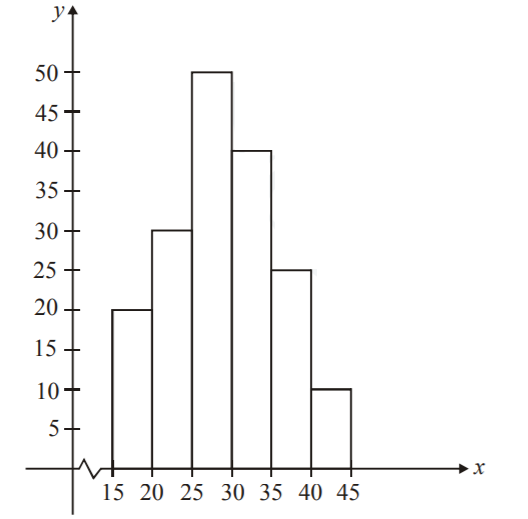Frequency polygon: